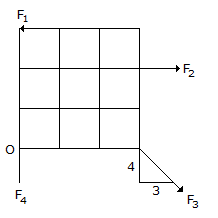# Civil Engineering - UPSC Civil Service Exam Questions

11.

A symmetrical channel section is made of a material which is equally strong in tension and compression. It is used as a simply supported beam with its web horizontal to carry vertical loads. It will

 A. be strongest if the web is used as top face B. be strongest if the web is used as bottom face C. be equally strong in (a) and (b) above D. not be possible to state which of the above statements is correct

Explanation:

No answer description available for this question. Let us discuss.

12.

A machine costing Rs. 8, 500 will have a scrap value of Rs. 300. Machines of this class have a working hour average life of 25, 000 hours. What will be the depreciation charge at the end of the first year, if the machine is operated for a total of 1500 hours ? (use straight line method of depreciation)

 A. Rs. 492.00 B. Rs. 542.00 C. Rs. 548.50 D. Rs. 692.00

Explanation:

No answer description available for this question. Let us discuss.

13.

The limits of percentage p of the longitudinal reinforcement in a column is

 A. 0.15% to 2% B. 0.8% to 4% C. 0.8% to 6% D. 0.8% to 8%

Explanation:

No answer description available for this question. Let us discuss.

14.

Figure shows the forces acting on a body. Each square is 3 cm x 3 cm. If F1 = 30 N, F2 = 15 N, F3 = 25 N and F4 = 20 N, the resultant force and the moment about point O, respectively, areA. 0 N, 0 Ncm B. 90 N, 80 Ncm C. 60 N, 60 Ncm D. 30 N, 30 Ncm

Explanation:

No answer description available for this question. Let us discuss.

15.

A simply supported beam of span (l) carries a point load W at midspan. The breadth 'b' of the beam along the entire span is constant. Given, f = permissible stress in bending, for a beam of uniform strength, the depth of the beam at any cross-section, at a distance x from the support would be :

 A. 6Wx/fb B. 6Wx/fb C. 3 Wx/fb D. 3 Wx/fb